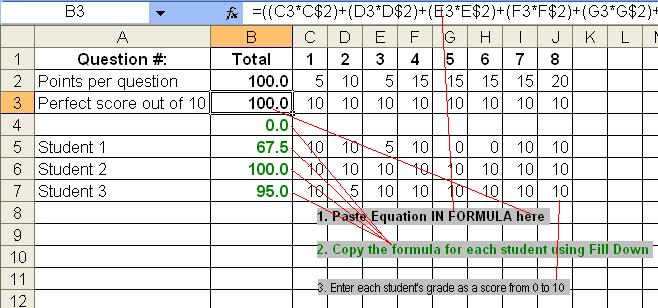From Column: To Column: Row: Weight Row:

Include Equals Sign

Divide byUsage: Using the above example, set up the question columns and then select the beginning and ending column. Press 'Make Excel Formula' and then

1. Copy the contents of the text area into the formula on the sheet as marked.
2. Fill down the formula for each student
3. Enter student grades at the intersection of the question and their name in a score from 0 to 10 and their total score will appear automatically

Note that you can use any scale you want if you change the amount you divide the entire equation by.